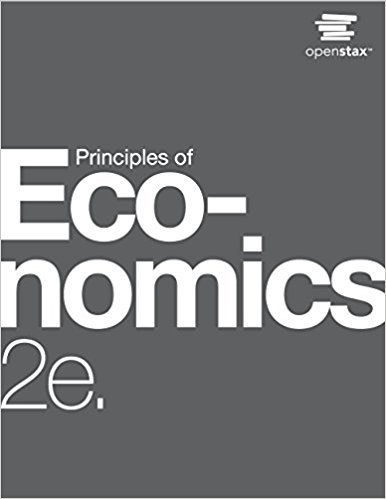×

×

# Solutions for Chapter 11: Monopoly and Antitrust Policy## Full solutions for Principles of Economics | 2nd Edition

ISBN: 9781947172364Solutions for Chapter 11: Monopoly and Antitrust Policy

Solutions for Chapter 11
4 5 0 338 Reviews
28
2
##### ISBN: 9781947172364

Principles of Economics was written by and is associated to the ISBN: 9781947172364. Since 39 problems in chapter 11: Monopoly and Antitrust Policy have been answered, more than 45776 students have viewed full step-by-step solutions from this chapter. This expansive textbook survival guide covers the following chapters and their solutions. This textbook survival guide was created for the textbook: Principles of Economics, edition: 2. Chapter 11: Monopoly and Antitrust Policy includes 39 full step-by-step solutions.

Key Business Terms and definitions covered in this textbook
• common resources

goods that are rival in consumption but not excludable

• diminishing marginal product

the property whereby the marginal product of an input declines as the quantity of the input increases

• economies of scale

the property whereby long-run average total cost falls as the quantity of output increases

• equilibrium price

the price that balances quantity supplied and quantity demanded

• excludability

the property of a good whereby a person can be prevented from using it

• explicit costs

input costs that require an outlay of money by the firm

• fixed costs

costs that do not vary with the quantity of output produced

• game theory

the study of how people behave in strategic situations

• implicit costs

input costs that do not require an outlay of money by the firm

• indexation

the automatic correction by law or contract of a dollar amount for the effects of inflation

• inflation tax

the revenue the government raises by creating money

• marginal cost

the increase in total cost that arises from an extra unit of production

• marginal product

the increase in output that arises from an additional unit of input

• median voter theorem

a mathematical result showing that if voters are choosing a point along a line and each voter wants the point closest to his most preferred point, then majority rule will pick the most preferred point of the median voter

• monetary neutrality

the proposition that changes in the money supply do not affect real variables

• perfect substitutes

two goods with straight-line indifference curves

• prisoners’ dilemma

a particular “game” between two captured prisoners that illustrates why cooperation is difficult to maintain even when it is mutually beneficial

• scarcity

the limited nature of society’s resources

• velocity of money

the rate at which money changes hands

• willingness to pay

the maximum amount that a buyer will pay for a good